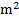# Point masses 1, 2, 3 and 4 kg are lying at the point (0, 0, 0), (2, 0, 0), (0, 3, 0) and (-2, -2, 0) respectively. The moment of inertia of this system about-axis will be a) 43 kg-b) 34 kg-c) 27 kg-d) 72 kg-## Question ID - 52684 :- Point masses 1, 2, 3 and 4 kg are lying at the point (0, 0, 0), (2, 0, 0), (0, 3, 0) and (-2, -2, 0) respectively. The moment of inertia of this system about-axis will be a) 43 kg-b) 34 kg-c) 27 kg-d) 72 kg-3537

(a)

Moment of inertia of the whole system about the axis of rotation will be equal to the sum of the moments of inertia of all  the particles.Next Question :
 The graph betweenand r( radial distance) is shown below. This represents(a) 1s orbital (b) 2s orbital (c) 2p orbital (d) 3s orbital